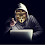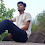# c++ break statement

In this article, you will learn about the break statement in the cpp language and usage of break statements in the loops.

The break statement breaks the execution of the loop in which it is used. Actually, break statements also use in the switch statement to break the execution in C++ language. The basic function of the break statement to stop the execution in the program.

### What is the syntax of the break statement?

The syntax of the break statement

break;

If you want to learn the usage of break statements, you must learn loops first.

## How break statement work?

Example break statement with for loop
//understand the break statement by
//displaying the counter of loop

#include<iostream>
using namespace std;

int main()
{
for(int i=0;i<5;i++)
{
//if loop counter reach at value of 3
//then loop terminate due to break staement
if(i==3)
{
break;//if a=3 then loop terminate
}
cout<<" value of counter = "<<i<<endl;

}
return 0;
}

output:
value of counter =0
value of counter =1
value of counter =2

In this example, the loop executes as usually but at the condition meet then break statement work.
If i=3 the loop execution stop, terminate the loop and do not print the counter of the loop because of the cout statement written after the break statement.

### How to use the break statement in the program of CPP?

Example using with a do-while loop
//take input number from the user
//until the user enters zero

#include<iostream>
using namespace std;

int main()
{
int a=5;            //declare variable a and define its value is five.
do
{
//take input from the user untill he
//press zero
cout<<" enter the number and enter zero for exist ";
cin>>a;
if(a==0)
{
break;            //if a=3 then loop terminate because of usage of a break.
}
cout<<a<<endl;
}while(true);
return 0;
}

Output
enter the number and enter zero for exist 1
1
enter the number and enter zero for exist -3
-3
enter the number and enter zero for exist 5
5
enter the number and enter zero for exist 0

In the example, the program takes input until the user enters zero and at the zero condition placed with the break statement in the loop. The break statement terminates the loop.

Example with a nested loop

The usage of break statement with the nested loop is interested in building the logic.

#include<iostream>
using namespace std;

int main()
{
for(int a=0;a<3;a++)
{
for(int b=0;b<3;b++)
{
if (b==2)
{
break;//inner loop terminate when b=2
}
cout<<" value of counter "<<a<<" "<<b<<endl;           //display the value of countera and counter b.
}
}
return 0;
}

output
value of counter 0 0
value of counter 0 1
value of counter 1 0
value of counter 1 1
value of counter 2 0
value of counter 2 1

In the above code, two nested for loops are used with the break statement. In the inner loop, display the value of the counter. If the counter value becomes two then the break statement terminates the inner loop and again returns toward the upper loop. Again upper loop starts its next iteration.

Please write the comment,if you find anything incorrect and any problem in the above topic or you want to share more information about the above topic.

1.Nice Content You Write A Professionall Article About Coding ANd Programing
I Also Write A Article About https://www.technicalmmub.gq/2020/07/top-10-programming-languages-in-2020.html
Top 10 Programming Languages in 2020 | Most Demanded Programming Languages

2.I'm love to program.. This is basic but very useful information. Stay motivated with Hindi Quotes

3.nice article sir .

4.Hi dear, Your post is really great! Thanks for mention.
Software store